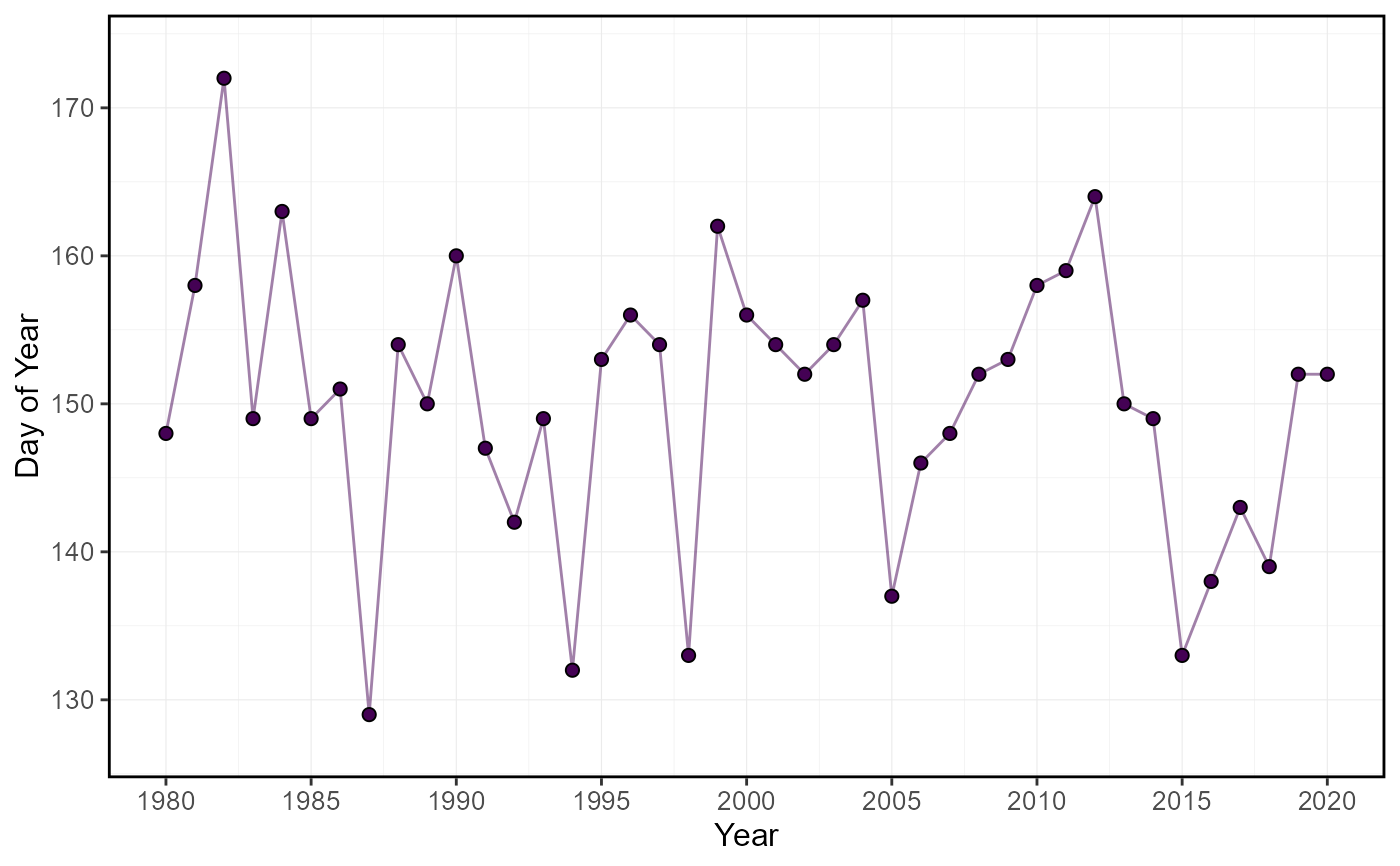Plots the timing (day of year and date) of portions of total annual flow of daily flow values from a daily streamflow data set. Calculates statistics from all values from complete years, unless specified. Data calculated using calc_annual_flow_timing() function. Returns a list of plots.

plot_annual_flow_timing(
data,
dates = Date,
values = Value,
groups = STATION_NUMBER,
station_number,
percent_total = c(25, 33.3, 50, 75),
water_year_start = 1,
start_year,
end_year,
exclude_years,
include_title = FALSE
)

## Arguments

data Data frame of daily data that contains columns of dates, flow values, and (optional) groups (e.g. station numbers). Leave blank if using station_number argument. Name of column in data that contains dates formatted YYYY-MM-DD. Only required if dates column name is not 'Date' (default). Leave blank if using station_number argument. Name of column in data that contains numeric flow values, in units of cubic metres per second. Only required if values column name is not 'Value' (default). Leave blank if using station_number argument. Name of column in data that contains unique identifiers for different data sets, if applicable. Only required if groups column name is not 'STATION_NUMBER'. Function will automatically group by a column named 'STATION_NUMBER' if present. Remove the 'STATION_NUMBER' column beforehand to remove this grouping. Leave blank if using station_number argument. Character string vector of seven digit Water Survey of Canada station numbers (e.g. "08NM116") of which to extract daily streamflow data from a HYDAT database. Requires tidyhydat package and a HYDAT database. Leave blank if using data argument. Numeric vector of percents of total annual flows to determine dates. Default c(25,33.3,50,75). Numeric value indicating the month (1 through 12) of the start of water year for analysis. Default 1. Numeric value of the first year to consider for analysis. Leave blank to use the first year of the source data. Numeric value of the last year to consider for analysis. Leave blank to use the last year of the source data. Numeric vector of years to exclude from analysis. Leave blank to include all years. Logical value to indicate adding the group/station number to the plot, if provided. Default FALSE.

## Value

A list of ggplot2 objects with the following for each station provided:

Annual_Flow_Timing

a plot that contains each n-percent of total volumetric discharge

Default plots on each object:
DoY_25pct_TotalQ

day of year of 25-percent of total volumetric discharge

DoY_33.3pct_TotalQ

day of year of 33.3-percent of total volumetric discharge

DoY_50pct_TotalQ

day of year of 50-percent of total volumetric discharge

DoY_75pct_TotalQ

day of year of 75-percent of total volumetric discharge

• Barnett, T.P., Pierce, D.W., Hidalgo, H.G., Bonfils, C., Santer, B.D., Das, T., Bala, G., Wood, A.W., Nozawa, T., Mirin, A.A., Cayan, D.R., Dettinger, M.D., 2008. Human-Induced Clanges in the Hydrology of the Western United States. Science 319, 1080-1083.

## See also

calc_annual_flow_timing

## Examples

# Run if HYDAT database has been downloaded (using tidyhydat::download_hydat())
if (file.exists(tidyhydat::hy_downloaded_db())) {

# Plot annual flow timing statistics with default percent totals
plot_annual_flow_timing(station_number = "08NM116")

# Plot annual flow timing with custom percent totals
plot_annual_flow_timing(station_number = "08NM116",
percent_total = 50)

}
#> Warning: One or more calculations included missing values and NA's were produced. Filter data for complete years or months, or use to ignore_missing = TRUE to ignore missing values.#> Warning: One or more calculations included missing values and NA's were produced. Filter data for complete years or months, or use to ignore_missing = TRUE to ignore missing values.#> \$Annual_Flow_Timing#>